# Getting fancy with ggplot2: code for alternatives to grouped bar charts

In this post I present the code I wrote to prodocue the figures in my previous post about alternatives to grouped bar charts.

Here I provide the code I used to create the figures from my previous post on alternatives to grouped bar charts. You are encouraged to play with them yourself!

The key to creating unique and creative visualizations using libraries such as ggplot (or even just straight SVG) is (1) to move away from thinking of data visualization only as the default plot types (bar plots, boxplots, scatterplots, etc), and (2) to realise that most visualizations are essentially lines and circles that you can arrange however you desire in space. Drawing a picture on paper before beginning to code your data viz is a great way to create customized visualizations for each dataset.

# Bars

First, I load in the libraries (the data comes from the dslabs library), and convert the data to long-form for creating the grouped bar charts.

# load in libraries
library(tidyverse)
library(dslabs)
# combine male and female success rates into single data frame
success_rates <- rbind(
# male success rates
transmute(research_funding_rates,
discipline,
success = success_rates_men,
gender = "Male"),
# female success rates
transmute(research_funding_rates,
discipline,
success = success_rates_women,
gender = "Female")) 
# print the data
success_rates
##             discipline success gender
## 1    Chemical sciences    26.5   Male
## 2    Physical sciences    19.3   Male
## 3              Physics    26.9   Male
## 4           Humanities    14.3   Male
## 5   Technical sciences    15.9   Male
## 6    Interdisciplinary    11.4   Male
## 7  Earth/life sciences    24.4   Male
## 8      Social sciences    15.3   Male
## 9     Medical sciences    18.8   Male
## 10   Chemical sciences    25.6 Female
## 11   Physical sciences    23.1 Female
## 12             Physics    22.2 Female
## 13          Humanities    19.3 Female
## 14  Technical sciences    21.0 Female
## 15   Interdisciplinary    21.8 Female
## 16 Earth/life sciences    14.3 Female
## 17     Social sciences    11.5 Female
## 18    Medical sciences    11.2 Female

I used geom_bar() to create a grouped bar chart containing the data. I grouped by gender by setting fill = gender within the aes() function.

# make grouped bar plot
ggplot(success_rates) +
# add bar for each discipline colored by gender
geom_bar(aes(x = discipline, y = success, fill = gender),
stat = "identity", position = "dodge") +
# name axes and remove gap between bars and y-axis
scale_y_continuous("Success Rate", expand = c(0, 0)) +
scale_x_discrete("Discipline") +
scale_fill_manual(values = c("#468189", "#9DBEBB")) +
# remove grey theme
theme_classic(base_size = 18) +
# rotate x-axis and remove superfluous axis elements
theme(axis.text.x = element_text(angle = 90,
hjust = 1, vjust = 0),
axis.line = element_blank(),
axis.ticks.x = element_blank())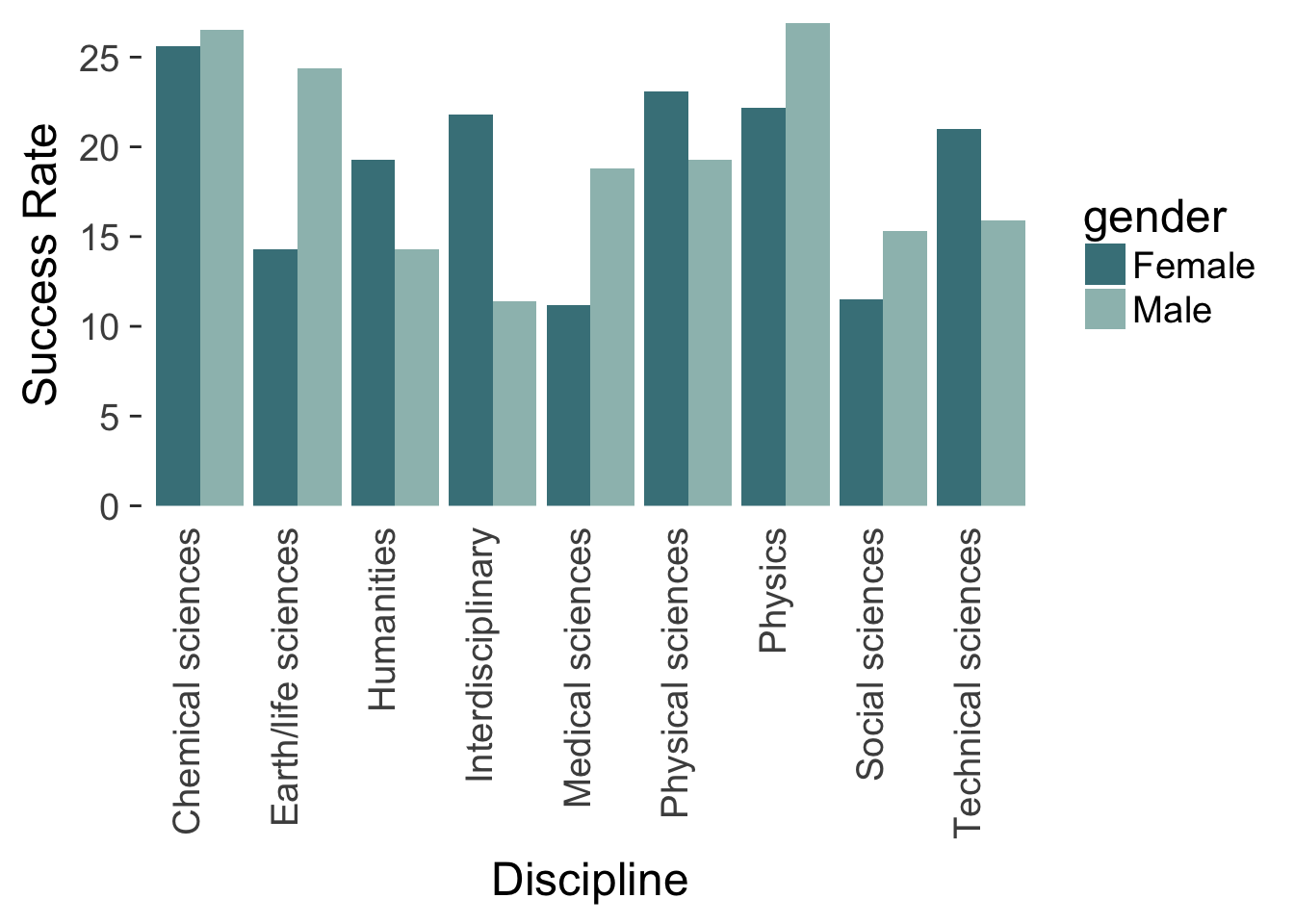# Slope plot

To create the sloped chart, it ends up being easier to keep the data in its original wide form.

The first element I add is the slopes from men to women using geom_segment(). Each slope is colored based on whether men or women are more successful.

gg_slope <- research_funding_rates %>%
# add a variable for when men are more successful than women (for colours)
mutate(men_more_successful = success_rates_men > success_rates_women) %>%
ggplot() +
# add a line segment that goes from men to women for each discipline
geom_segment(aes(x = 1, xend = 2,
y = success_rates_men,
yend = success_rates_women,
group = discipline,
col = men_more_successful),
size = 1.2) +
# set the colors
scale_color_manual(values = c("#468189", "#9DBEBB"), guide = "none")  +
# remove all axis stuff
theme_classic() +
theme(axis.line = element_blank(),
axis.text = element_blank(),
axis.title = element_blank(),
axis.ticks = element_blank())
gg_slope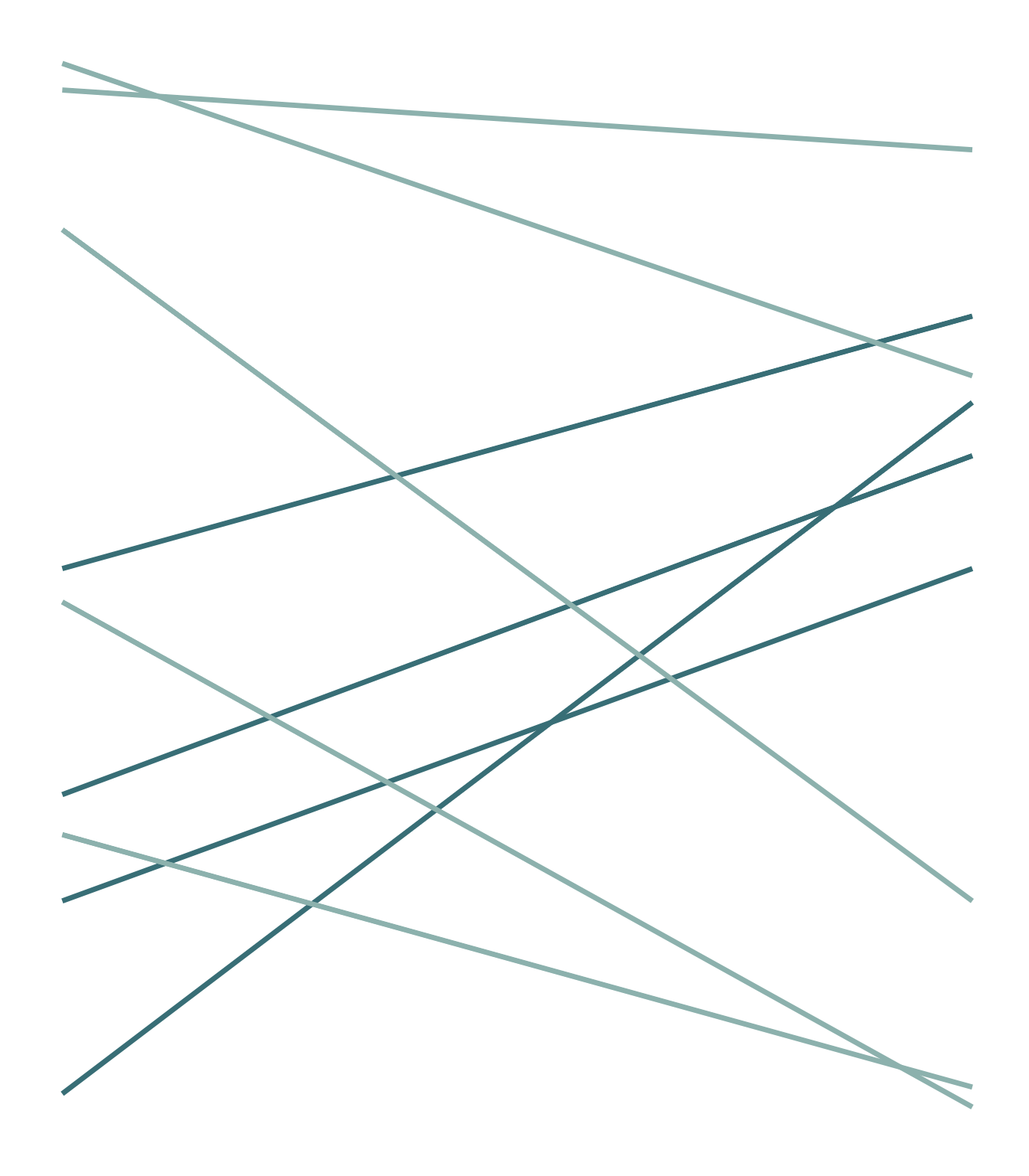Next, I add a left and right axis at the start and end of the slopes.

gg_slope <- gg_slope +
# add vertical lines that act as axis for men
geom_segment(x = 1, xend = 1,
y = min(research_funding_rates$success_rates_men) - 2, yend = max(research_funding_rates$success_rates_men) + 1,
col = "grey70", size = 0.5) +
# add vertical lines that act as axis for women
geom_segment(x = 2, xend = 2,
y = min(research_funding_rates$success_rates_men) - 2, yend = max(research_funding_rates$success_rates_men) + 1,
col = "grey70", size = 0.5) +
# add the words "men" and "women" above their axes
geom_text(aes(x = x, y = y, label = label),
data = data.frame(x = 1:2,
y = 2 + max(research_funding_rates\$success_rates_men),
label = c("men", "women")),
col = "grey30",
size = 6)
gg_slope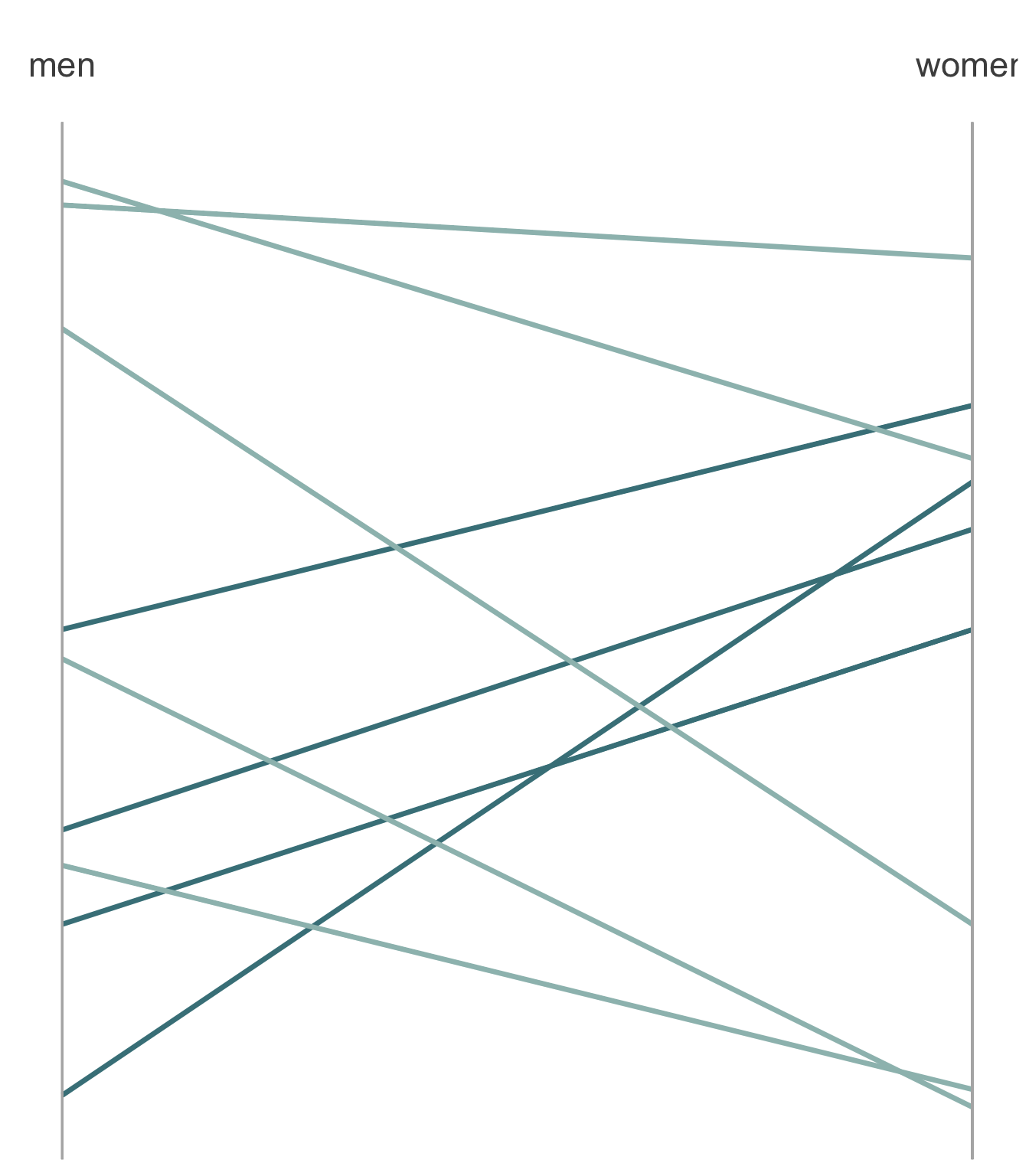I also need to add an identifier for each discipline’s slope. I do this by annotating the plot with text.

gg_slope <- gg_slope +
# add the label and success rate for each discipline next the men axis
geom_text(aes(x = 1 - 0.03,
y = success_rates_men,
label = paste0(discipline, ", ",
round(success_rates_men, 1), "%")),
col = "grey30", hjust = "right") +
# add the success rate next to each point on the women axis
geom_text(aes(x = 2 + 0.08,
y = success_rates_women,
label = paste0(round(success_rates_women, 1), "%")),
col = "grey30") +
# set the limits of the x-axis so that the labels are not cut off
scale_x_continuous(limits = c(0.5, 2.1))
gg_slope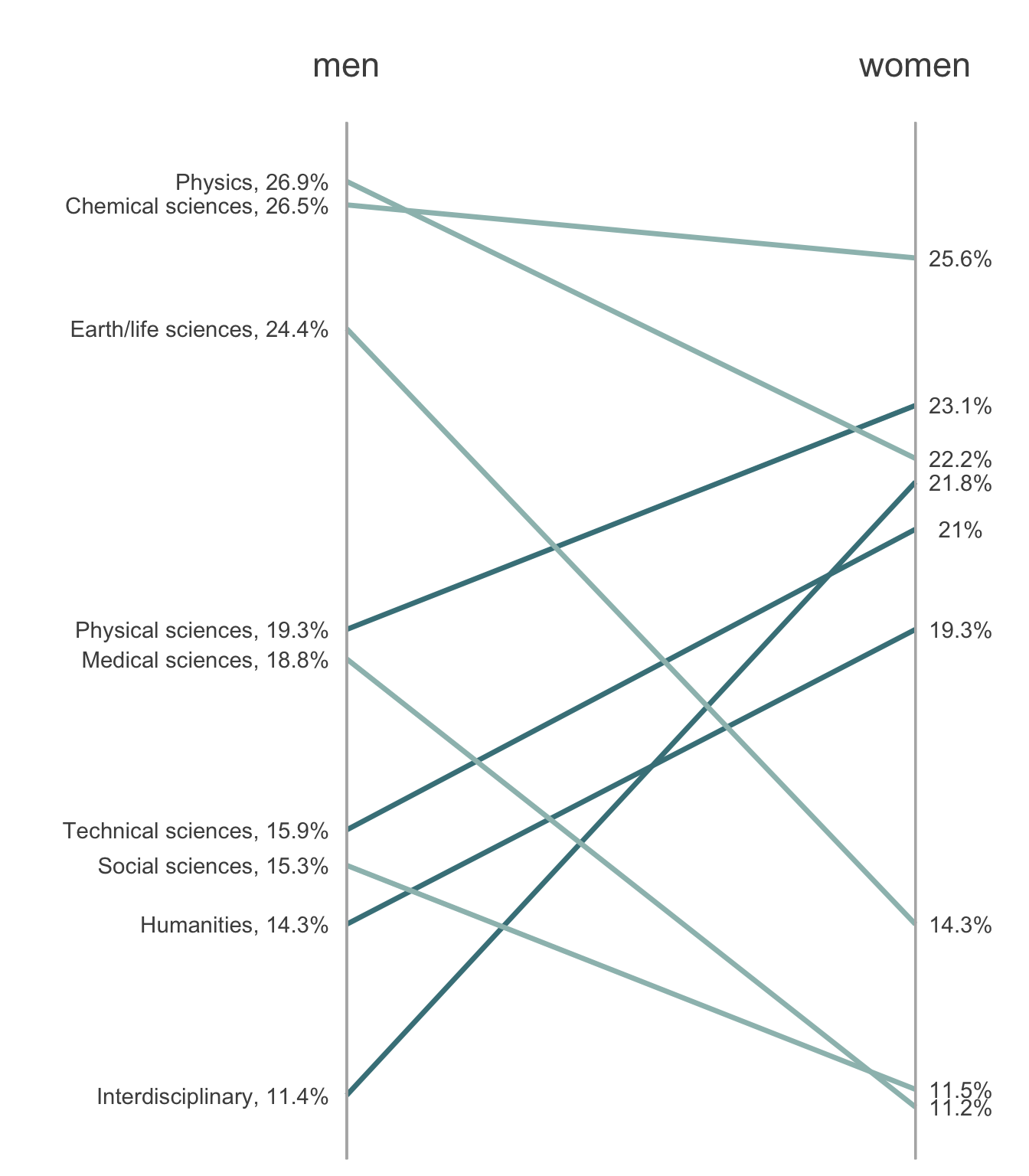Finally, I decide to add points (for aesthetic purposes). Behind each circle, I add a slightly larger white circle to act as a border and to give a slight “gap” look.

gg_slope <- gg_slope +

# add the white outline for the points at each rate for men
geom_point(aes(x = 1,
y = success_rates_men), size = 4.5,
col = "white") +
# add the white outline for the points at each rate for women
geom_point(aes(x = 2,
y = success_rates_women), size = 4.5,
col = "white") +

# add the actual points at each rate for men
geom_point(aes(x = 1,
y = success_rates_men), size = 4,
col = "grey60") +
# add the actual points at each rate for men
geom_point(aes(x = 2,
y = success_rates_women), size = 4,
col = "grey60")
gg_slope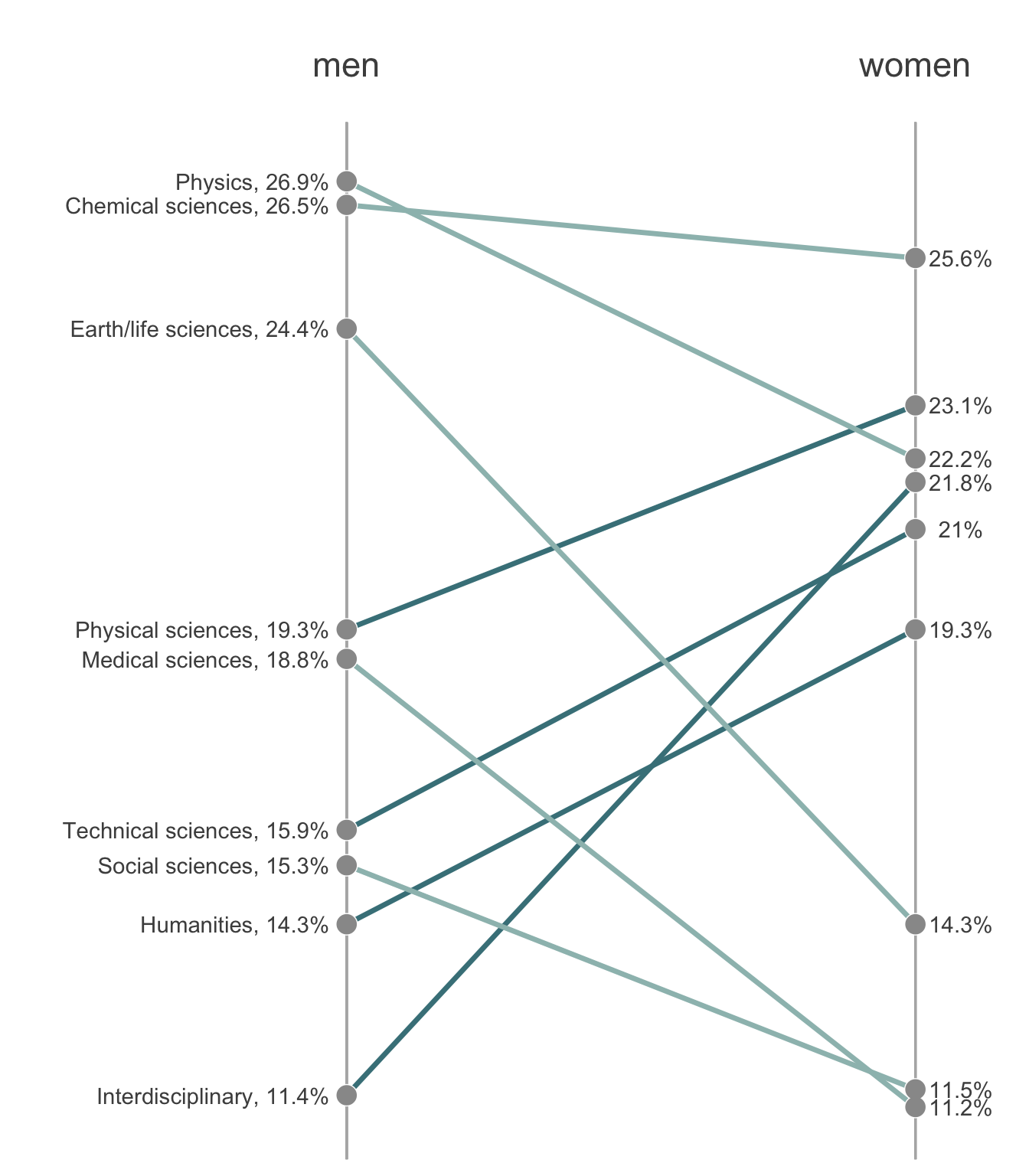# Horizontal dots

To create the horizontal dot plot, I again keep the data in its original wide-form but I arrange the disciplines in order of women’s success rate (this will make the plot easier to read).

The first things I do to create the dot plot is add the horizontal discipline lines and the points for the success rates of men and women.

gg_dot <- research_funding_rates %>%
# rearrange the factor levels for discipline by rates for women
arrange(success_rates_women) %>%
mutate(discipline = fct_inorder(discipline)) %>%

ggplot() +
# remove axes and superfluous grids
theme_classic() +
theme(axis.title = element_blank(),
axis.ticks.y = element_blank(),
axis.line = element_blank()) +

# add a dummy point for scaling purposes
geom_point(aes(x = 12, y = discipline),
size = 0, col = "white") +

# add the horizontal discipline lines
geom_hline(yintercept = 1:9, col = "grey80") +

# add a point for each male success rate
geom_point(aes(x = success_rates_men, y = discipline),
size = 11, col = "#9DBEBB") +
# add a point for each female success rate
geom_point(aes(x = success_rates_women, y = discipline),
size = 11, col = "#468189")
gg_dotSince I really like to annotate my figures with text so that the audience can work less hard, I add the success rate on top of each dot. I also prefer to annotate features my plot directly instead of using legends, so I also add a label for men and women.

gg_dot <- gg_dot +
# add the text (%) for each male success rate
geom_text(aes(x = success_rates_men, y = discipline,
label = paste0(round(success_rates_men, 1))),
col = "black") +
# add the text (%) for each female success rate
geom_text(aes(x = success_rates_women, y = discipline,
label = paste0(round(success_rates_women, 1))),
col = "white") +
# add a label above the first two points
geom_text(aes(x = x, y = y, label = label, col = label),
data.frame(x = c(25.6 - 1.1, 26.5 + 0.6), y = 10,
label = c("women", "men")), size = 6) +
scale_color_manual(values = c("#9DBEBB", "#468189"), guide = "none") +

# manually specify the x-axis
scale_x_continuous(breaks = c(15, 20, 25),
labels = c("15%", "20%", "25%")) +
# manually set the spacing above and below the plot
scale_y_discrete(expand = c(0.2, 0))
gg_dot